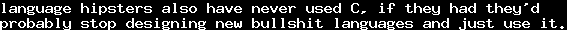# A Wrong Construction of a Field With One ElementThu, 06 Oct 2016 14:37:04 GMT

As I don't really know where else to publish this (mathoverflow etc do not seem to have a section for it), here comes a wrong construction of a field of characteristic 1. Still, it is wrong in a somewhat interesting way. Maybe as an exercise for an introductory lecture in mathematical logic or something.

So to make things easy, we work with ZFC. We could probably use weaker systems, but this would just make things harder to express.

Now, letbe the first-order theory of Zermelo-Fraenkel set theory, and let us assume that. We define first-order constants,,,,with the axioms

•••is a field

Now defineand, where the first addition is ordinal addition, and the second addition is addition in the field. Essentially, this defines thatmeans addingfortimes. This is a simple recursive definition, so it is justified according to the recursion theorem. We add the axiom

•(has finite characteristic)

let us call the resulting theory. Obviously,. Now define the axioms, and, and consider.

Every finite subset ofis satisfiable, because there are fields of arbitrarily large characteristic. Hence, because of the compactness theorem,, and hence, there is a modelwith a constantsuch that

•is a field
•has finite characteristic
•for all.

We can "lift"out of its model, by setting, similar for the other constants.

Now comes the exercise: Why isnot a field of characteristic 1?

I could actually imagine that, though the actual characteristic ofshould be 0 (if I am correct), this thing has some properties that one wants from fields with characteristic 1, so it might be interesting to look at (but if it is, there certainly are people who already do this).